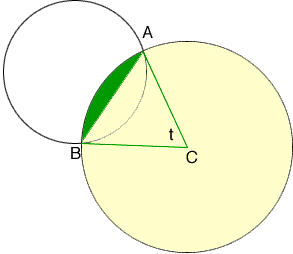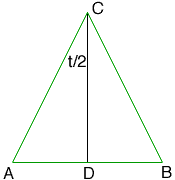Quandaries and Queries I Have a problem to calculate the area of two overlapping circles because two circles are overlap then how i calculate the overlap area to subtract from the area of both circle. Hi, I drew a diagram and labeled some points. I want to focus on the larger of the two circles and find the area of part of the overlap, the piece shaded green in the diagram. The area of the remainder of the overlap can be found by a simpler calculation using the smaller of the two circles.The center of the circle is C. If you know t, the measure of the angle BCA in radians and the radius r of the circle then the sector of ABC of the circle has area given by sector ABC area = 1/2r2t The green piece of the overlap can then be found by subtracting the area of the triangle ABC from the area of the sector ABC.The length of CA is the radius r of the circle and the height h of the triangle is the length of CD. But cos( t/2) = |CD|/|CA| = h/r Thus h = rcos( t/2). Likewise |AD| = rsin( t/2) and the area of the triangle ABD is |AD|h. Penny Go to Math Central